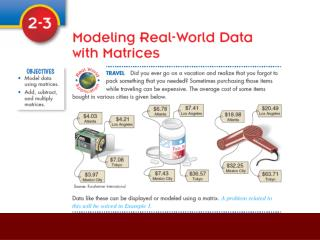DownloadDownload PresentationMatrix

# Matrix

Télécharger la présentation## Matrix

- - - - - - - - - - - - - - - - - - - - - - - - - - - E N D - - - - - - - - - - - - - - - - - - - - - - - - - - -
##### Presentation Transcript

1. Matrix A rectangular array of terms. Each term is an element of the matrix. Each matrix is describe by its dimensions. ( m x n) Where m is the number of rows and n is the number of columns.

2. Types of Matrices Row matrix – contains only one row Column matrix – contains only one column Square matrix – has the same number of rows as columns, sometimes called an nth order matrix, where n is the number of rows and columns Elements can be represented by a double subscript. Aij represents the element in the ith row and the jth column.

3. Example 1 a. b. Locate the price of the pain reliever in Mexico. (Row 2, Column 3) a23 (a is an arbitrary letter)

4. Equal Matrices Two matrices are equal if and only if they have the same dimensions and are identical, element by element. Remember: If and only if means the converse is also true. Write the converse: If two matrices have the same dimensions and are identical, element by element, then they are equal.

5. Example 2 If two matrices are equal, their corresponding elements are equal, so . . . Select a method to solve: graphing, substitution, elimination. substitution X = 2(2) = 4 Solution: (4, 2)

6. Addition of Matrices The sum of two m x n matrices is an m x n matrix in which the elements are the sum of the corresponding elements of the given matrices. • The matrices have to be the same dimensions • The resulting matrix is also the same dimension • The elements of the resulting matrix is derived from adding the corresponding elements

7. Example 3

8. Zero Matrix – Identify Matrix An m x n matrix where all elements are equal to zero. Additive Identity – the number that can be added to anything resulting in no change

9. Subtraction of Matrices The difference A – B of two m x n matrices is equal to the sum A + (-B), where –B represents the additive inverse of B. Remember: Any subtraction problem can be rewritten as an addition problem. “Add the Opposite”

10. Example 4

11. Scalar Product The product of scalar k and an m x n matrix A is an m x n matrix denoted by kA. Each element of kA equals k times the corresponding element of A.

12. Example 5

13. Product of Two Matrices The product of an m x n matrix A and an n x r matrix B is an m x r matrix AB. The ijth element of AB is the sum of the products of the corresponding elements in the ith row of A and the jth column of B. • Not all matrices can be multiplied. • The number of columns in the first matrix must match the number of rows in the second matrix. • The resulting product’s dimension is the number of rows as the first matrix by the number of columns of the second matrix. • The resulting elements – Let me show you. It’s easier to understand that way!

14. Multiplying Matrices In order to multiply matrices, the number of columns in the first matrix must match the number of rows in the second matrix. (Always check first, otherwise you may be wasting your time!) 3 x 3 2 x 3 Resulting matrix: 2 x 3 The dimensions of the resulting matrix will be the number of rows from the first matrix and the number of columns of the second matrix.

15. Multiplying Matrices 30 x 45 x 30 x 30 x 45 x 30 x 30 x 45 x 30 x + + + + + + 28 x 32 x 25 x 28 x 32 x 25 x 28 x 32 x 25 x Drop! Bump! Bump! 30(1)+45(0.7)+30(0.9) 30(0)+45(0.4)+30(0) 30(0)+45(0)+30(0.15) Bump! Stop! Bump! 28(0)+32(0.4)+25(0) 28(1)+32(0.7)+25(0.9) 28(0)+32(0)+25(0.15) [ ] 88.5 18 4.5 72.9 12.8 3.75

16. Example 6 2 x 3 2 x 2 Is it possible to multiply AB? Is it possible to multiply BC? 2 x 3 2 x 3

17. Example 7 Be sure to create the matrix multiplication that can be completed.

18. HW : Page 82 (5– 31 odds)